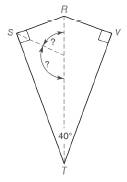Chapter 4.2, Problem 40EElementary Geometry For College St...

7th Edition
Alexander + 2 others
ISBN: 9781337614085

Solutions

Chapter
SectionElementary Geometry For College St...

7th Edition
Alexander + 2 others
ISBN: 9781337614085
Textbook Problem

R S T V is a kite, with R S ¯ ⊥ S T ¯ and R V ¯ ⊥ V T ¯ . If m ∠ S T V = 40 ° , how large is the angle formeda) by the bisectors of ∠ R S T and ∠ S T V ? b) by the bisectors of ∠ S R V and ∠ R S T ?To determine

a)

The angle formed by the bisectors of RST and STV.

Explanation

Calculation:

Given,

RSTV is a kite

RS¯ST¯

RV¯VT¯

mSTV=40°

Let the bisectors of RST and STV intersect at ‘O’.

Now, we need to find the angle formed by the bisectors OS and OT, which is TOS.

In the kite T and S are bisected, thus in TOS,

OST=90°2=45°, and

STO=40°2=20°

To determine

b)

The angle formed by the bisectors of SRV and RST.

Still sussing out bartleby?

Check out a sample textbook solution.

See a sample solution

The Solution to Your Study Problems

Bartleby provides explanations to thousands of textbook problems written by our experts, many with advanced degrees!

Get Started

Differentiate the function. H(z)=a2z2a2+z2

Single Variable Calculus: Early Transcendentals, Volume I

What is the value of (X + 1) for the following scores: 0, 1, 4, 2? a. 8 b. 9 c. 11 d. 16

Essentials of Statistics for The Behavioral Sciences (MindTap Course List)

(1252/3)1/2

Applied Calculus for the Managerial, Life, and Social Sciences: A Brief Approach

43. What is the domain of Check with a graphing utility.

Mathematical Applications for the Management, Life, and Social Sciences

True or False: is a convergent series.

Study Guide for Stewart's Multivariable Calculus, 8th

2 1 0 does not exist

Study Guide for Stewart's Single Variable Calculus: Early Transcendentals, 8th